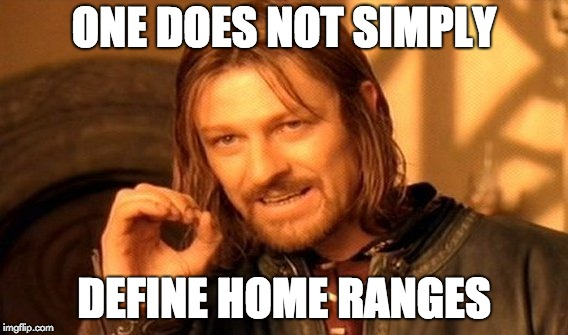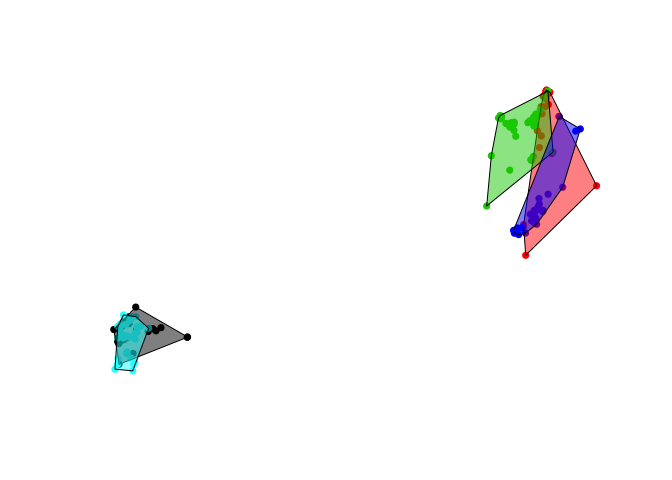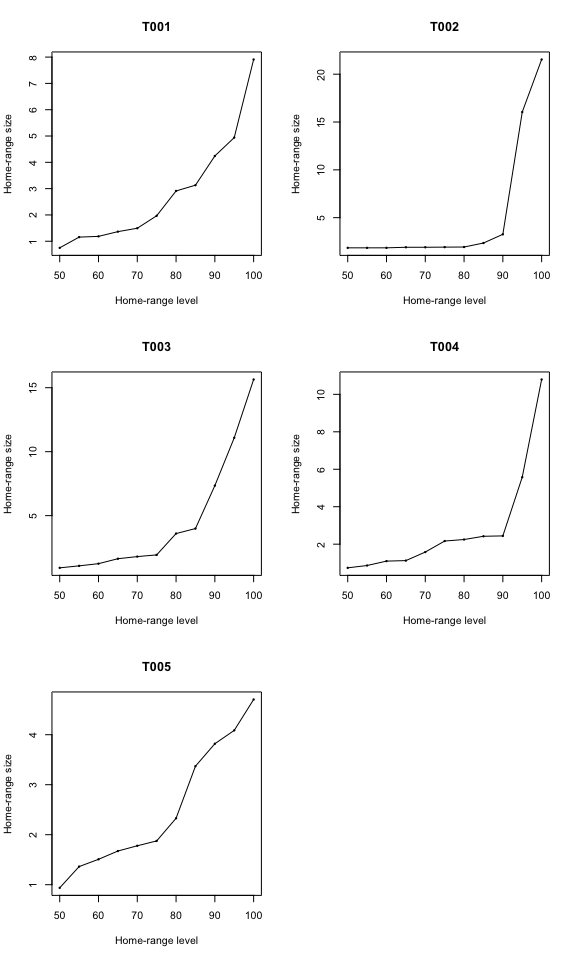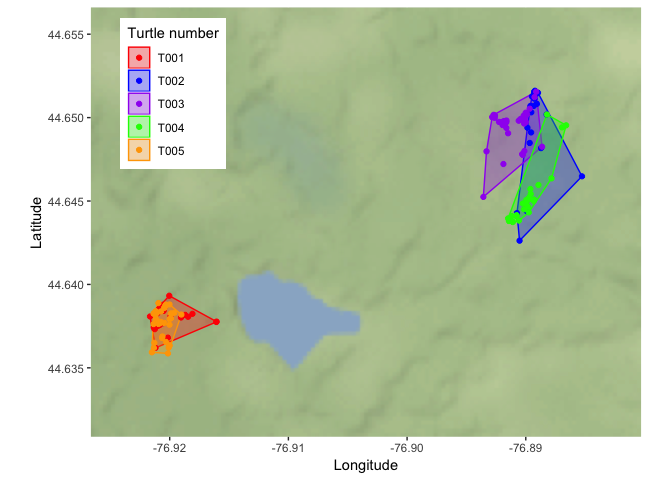Update 2020-04-02 I have updated the `ggmap` section to account for Google’s change in API. The tutorial now uses Stamen tiles, but I included code for downloading Google tiles and a link on how to register for a key.

What is a home range?

“that area traversed by the animal during its normal activities of food gathering, mating and caring for young. Occasional sallies outside the area, perhaps exploratory in nature, should not be considered as in part of the home range.” -Burt 1943

The most commonly cited definition is vague and does not provide a clear method for estimating the home range. So what should someone wanting to calculate home ranges do?

I will focus on two common methods to measure home ranges:

• Minimum convex polygons (here), and
• Kernel density estimators (next post)

The minimum convex polygon (MCP) draws the smallest polygon around points with all interior angles less than 180 degrees. MCPs are common estimators of home range, but can potentially include area not used by the animal and overestimate the home range.

Let’s use the `mcp` function from the `adehabitatHR` package on an example data set. The code and data used are available on my GitHub page.

``````# Read the csv file
turtles <- read.csv("tracking_sample.csv",
stringsAsFactors = FALSE)
# The file should be in your working directory.``````

To make MCP’s in R using the `adehabitatHR` package, the point layer should be a SpatialPointsDataframe. See my previous post on formatting telemetry data for spatial analyses.

``````# SpatialPointsDataFrame objects don't like missing values
# Remove two rows with NA's
turtles <- turtles[!is.na(turtles\$x) & !is.na(turtles\$y),]

# Only include three columns (id, x, and y coordinates) for making MCP's
turtles.sp <- turtles[, c("id", "x", "y")]

# Create a SpatialPointsDataFrame by defining the coordinates
library(sp)
coordinates(turtles.sp) <- c("x", "y")

# Set the coordinate reference system (CRS)
# More information on CRS here:
# https://www.nceas.ucsb.edu/~frazier/RSpatialGuides/OverviewCoordinateReferenceSystems.pdf
# The sample data are UTM points in WGS84 from zone 18N
proj4string(turtles.sp) <- CRS( "+proj=utm +zone=18 +datum=WGS84 +units=m +no_defs" )``````
``library(adehabitatHR) # Load library``
``````# Calculate MCPs for each turtle
turtles.mcp <- mcp(turtles.sp, percent = 100)

# Examine output
turtles.mcp``````
``````## Object of class "SpatialPolygonsDataFrame" (package sp):
##
## Number of SpatialPolygons:  5
##
## Variables measured:
##        id     area
## T001 T001  7.90870
## T002 T002 21.53665
## T003 T003 15.64180
## T004 T004 10.79325
## T005 T005  4.70450``````

We can see that `turtles.mcp` is a SpatialPolygonsDataFrame with one polygon per individual. Conveniently, the function also calculated the area. Since the input file used UTM, the area is in hectares by default.

You can change the area unit with the `unout` argument. Type `help(mcp)` for more details.

Let’s quickly plot our MCPs overtop of the points.

``````# Plot
library(scales) # Helps make polygons partly transparent using the alpha argument below
plot(turtles.sp, col = as.factor(turtles.sp@data\$id), pch = 16)
plot(turtles.mcp, col = alpha(1:5, 0.5), add = TRUE)``````In our original call to `mcp`, the percent argument was set to 100. Therefore, all of the relocations were used to construct the home range of each turtle. However, are all of these areas normally used by individuals?

We can easily examine how home range size changes by excluding a certain percentage of points using the `mcp.area` function. Let’s see how home range size changes by including 50 to 100 percent of points.

``````# Calculate the MCP by including 50 to 100 percent of points
hrs <- mcp.area(turtles.sp, percent = seq(50, 100, by = 5))````````hrs # examine dataframe``
``````##        T001     T002     T003     T004    T005
## 50  0.74950  1.84625  0.92010  0.73635 0.93695
## 55  1.15710  1.84625  1.07565  0.86125 1.36220
## 60  1.18535  1.84765  1.24635  1.09525 1.50770
## 65  1.36515  1.90495  1.63215  1.12310 1.67315
## 70  1.49430  1.90620  1.79935  1.57855 1.77820
## 75  1.96850  1.92045  1.93305  2.16755 1.87555
## 80  2.91200  1.93120  3.59960  2.24845 2.32870
## 85  3.13090  2.34895  3.99060  2.42160 3.37040
## 90  4.24175  3.26080  7.34455  2.44170 3.81925
## 95  4.94060 16.04270 11.08685  5.57750 4.08660
## 100 7.90870 21.53665 15.64180 10.79325 4.70450``````

We can see that T002, T003, and T004 in particular have mostly stable home range sizes with just a few outliers because the line is very steep between 90 and 100%. However, the line increases with more points almost linearly for T001 and T005.

Determining whether it is appropriate to exclude some points in estimating the home range will depend on your data, the biology of the species, and the definition of home range you choose. Whatever your choice, make sure you have evidence to back it up. With reptiles that typically move much less than many birds and mammals, usually all points are included when estimating home range size.

As a final exercise, let’s plot the MCP’s and points overtop a basemap using `ggmap`. Before we get started on making a map, we need to convert our SpatialPointsDataFrames and SpatialPolygonsDataFrames into latitude and longitude for `ggmap` using the `spTransform` function.

``````# Transform the point and MCP objects.
turtles.spgeo <- spTransform(turtles.sp, CRS("+proj=longlat"))
turtles.mcpgeo <- spTransform(turtles.mcp, CRS("+proj=longlat"))

# Download tiles using ggmap
library(ggmap)

# Google tiles (requires a key first)
## Guide on getting a key: https://www.r-bloggers.com/geocoding-with-ggmap-and-the-google-api/
# register_google(key = "mykeyhere")
# mybasemap <- get_map(location = c(lon = mean(turtles.spgeo@coords[,1]),
#                                   lat = mean(turtles.spgeo@coords[,2])),
#                      source = "google",
#                      zoom = 14,
#                     maptype = 'satellite')

mybasemap <- get_stamenmap(bbox = c(left = min(turtles.spgeo@coords[,1])-0.005,
bottom = min(turtles.spgeo@coords[,2])-0.005,
right = max(turtles.spgeo@coords[,1])+0.005,
top = max(turtles.spgeo@coords[,2])+0.005),
zoom = 12)

# Turn the spatial data frame of points into just a dataframe for plotting in ggmap
turtles.geo <- data.frame(turtles.spgeo@coords,
id = turtles.spgeo@data\$id )

mymap.hr <- ggmap(mybasemap) +
geom_polygon(data = fortify(turtles.mcpgeo),
# Polygon layer needs to be "fortified" to add geometry to the dataframe
aes(long, lat, colour = id, fill = id),
alpha = 0.3) + # alpha sets the transparency
geom_point(data = turtles.geo,
aes(x = x, y = y, colour = id))  +
theme(legend.position = c(0.15, 0.80)) +
labs(x = "Longitude", y = "Latitude") +
scale_fill_manual(name = "Turtle number",
values = c("red", "blue", "purple", "green", "orange"),
breaks = c("T001", "T002", "T003", "T004", "T005")) +
scale_colour_manual(name = "Turtle number",
values = c("red", "blue", "purple", "green", "orange"),
breaks = c("T001", "T002", "T003", "T004", "T005"))
mymap.hr``````Pretty neat, and simple! From examining the plot of MCPs above, you can see that some polygons include large areas not used by an individual. One solution to this problem is to create home ranges based on the density of points.

In the next post I will create home ranges using kernel density estimators.